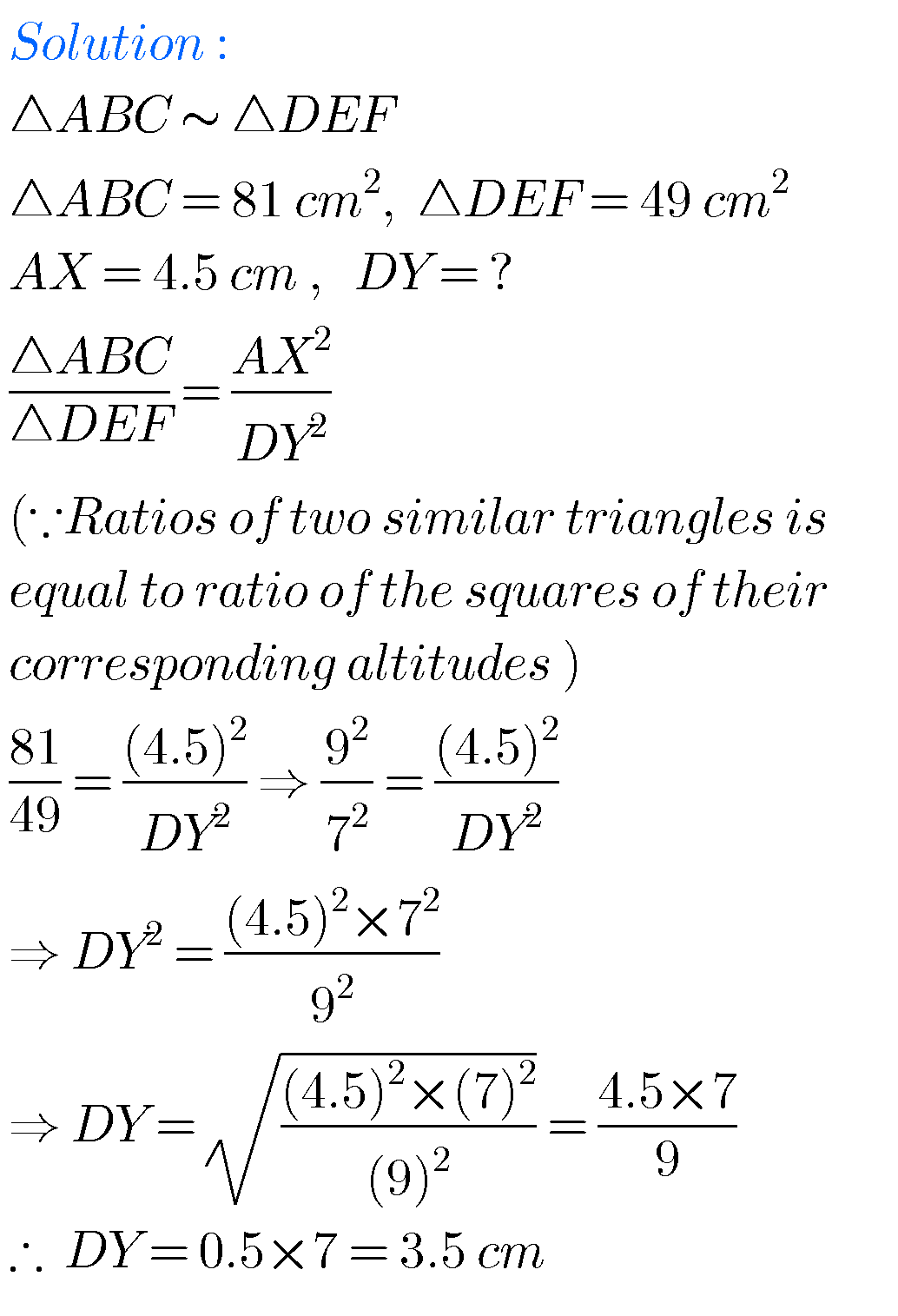## SSC solutions for Maths Similar Triangles class 10

SSC solutions for maths Similar Triangles class 10 Mathematics class 10 SSC Andhra predesh and Telangana Similar Triangles  exercise 8.1, exercise 8.2, exercise 8.3 and exercise 8.4  solutions are given. These solutions are very easy to understand. First you study the text book lesson. Observe the example problems and solutions in the text book. Try …